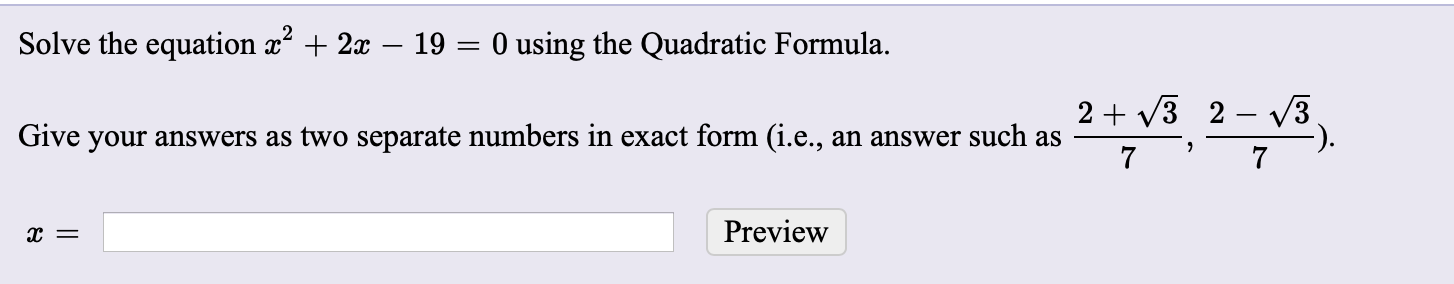# Solve the equation x + 2x – 19 = 0 using the Quadratic Formula.2 + V3 2 – v3Give your answers as two separate numbers in exact form (i.e., an answer such as7Preview

Question
2 viewshelp_outlineImage TranscriptioncloseSolve the equation x + 2x – 19 = 0 using the Quadratic Formula. 2 + V3 2 – v3 Give your answers as two separate numbers in exact form (i.e., an answer such as 7 Preview fullscreen
check_circle

Step 1

Compare the given equation with a...

### Want to see the full answer?

See Solution

#### Want to see this answer and more?

Solutions are written by subject experts who are available 24/7. Questions are typically answered within 1 hour.*

See Solution
*Response times may vary by subject and question.
Tagged in
MathAlgebra

### Other